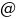﻿ Homepage of Slaven Kožić
English Hrvatski

# Slaven Kožić

Assistant Professor
Department of Mathematics
Faculty of Science
University of Zagreb
Bijenička cesta 30
10000 Zagreb, Croatia
E-mail: kslavenmath.hr

Research Teaching CV

## Research interests

My research interests are in the areas of quantum groups, infinite dimensional Lie algebras and vertex algebras.

## Papers and preprints

1. M. Butorac, S. Kožić, Principal subspaces for the quantum affine vertex algebra in type A1(1), arXiv:2011.13072 [math.QA].
2. M. Butorac, S. Kožić, M. Primc, Parafermionic bases of standard modules for affine Lie algebras, Math. Z. (2020), https://doi.org/10.1007/s00209-020-02639-w; arXiv:2002.00435 [math.QA].
3. S. Kožić, On the quantum affine vertex algebra associated with trigonometric R-matrix, arXiv:1908.06517 [math.QA].
4. M. Butorac, N. Jing, S. Kožić, h-Adic quantum vertex algebras associated with rational R-matrix in types B, C and D, .
5. M. Butorac, S. Kožić, Principal subspaces for the affine Lie algebras in types D, E and F, arXiv:1902.10794 [math.QA].
6. S. Kožić, Quantum current algebras associated with rational R-matrix, .
7. S. Kožić, Quasi modules for the quantum affine vertex algebra in type A, .
8. S. Kožić, Commutative operators for double Yangian DY(sln), Glas. Mat. Ser. III Vol. 53, No. 1 (2018), 97-113.
9. S. Kožić, Principal subspaces for double Yangian DY(sl2), J. Lie Theory 28 (2018), No. 3, 673-694.
10. S. Kožić, A. Molev, Center of the quantum affine vertex algebra associated with trigonometric R-matrix, .
11. S. Kožić, Higher level vertex operators for Uq(ŝl2), .
12. N. Jing, S. Kožić, A. Molev, F. Yang, Center of the quantum affine vertex algebra in type A, .
13. S. Kožić, Vertex operators and principal subspaces of level one for Uq(ŝl2), .
14. S. Kožić, A note on the zeroth products of Frenkel-Jing operators, .
15. S. Kožić, M. Primc, Quasi-particles in the principal picture of ŝl2 and Rogers-Ramanujan-type identities, .
16. S. Kožić, Principal subspaces for quantum affine algebra Uq(An(1)), .